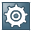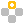ABC AnalysisUse

ABC analysis groups objects within a list according to their significance in regard to a particular numerical key figure.

ABC analysis is available with single-level lists if the list has at least one key figure.

Features

ABC analysis allows the grouping of objects in three segments:

• A segment = important objects
• B segment = less important objects
• C segment = relatively unimportant objectsYou use ABC analysis in order selection in Product Cost Controlling (CO-PC) to receive an overview of the production orders in a plant that incur the most actual costs. In the A segment you can see the orders that incurred the highest costs and that represent 50% of the entire actual costs in a plant. In the B segment you can see the orders with the next-lowest actual costs; up to 80% of the total costs of the plant are now reached. The orders that incurred low costs are shown in the C segment.

The object to be grouped must have a value for at least one key figure. In ABC analysis the objects are first sorted in ascending or descending order with reference to a preselected key figure. Then the list is subdivided using a predefined strategy. The following strategies can be used for grouping:

• Key figure (percentage)

The division into groups is based on the percentage shares of the key figures on the total value. For example, if the selected grouping is A = 50%, B = 30% and C = 20%, the objects in the list are sorted in ascending or descending order according to the value of the selected key figure and then assigned to segment A if the percentage share of the total of the key figures of the total value does not exceed 50%. Then the objects are assigned to the B segment if the percentage share of the total of the key figures does not exceed 80% of the total value. The remaining objects are assigned to the C segment.

• Key figure (absolute)

The division into groups is based on the absolute values of the key figures. Two limiting values must be specified: the limiting value between the A and B segments and the limiting value between the B and C segments. The objects are assigned to groups using these limiting values.

• Characteristics (percentage)

The division into groups is based on the percentage number of objects in the list compared to the total number of objects. For example, if the selected grouping is A = 50%, B = 30% and C = 20%, the objects in the list are first sorted in ascending or descending order according to the value of the selected key figure. The A segment therefore receives 50% of the total number of objects with the highest or lowest key figure value. The B segment receives the next 30% of the objects, and all remaining objects are assigned to the C segment.

• Characteristics (absolute)

The number of objects to be assigned to the A and B segments is specified by the user. The remaining objects are assigned to the C segment.

Activities

To perform ABC analysis:

1. Select a key figure column in the list to be used as the criteria for ABC analysis by positioning the cursor on the column header.
2. Choose ABC analysis. A dialog box appears showing the key figure you selected that is used as the basis for ABC analysis. The characteristics of the columns that are in the list to the left of the selected key figure column.
3. Choose the type of sorting (ascending or descending) and the strategy for grouping. Specify values for the grouping within the selected strategy.
4. Choose Enter.

Result

A list is displayed with the segment (A, B, or C) in the first column. This is followed by the object and further characteristics (such as the order type) and the selected key figure. After each key figure the percentage share of that key figure on the total is shown, along with the cumulative percentage share. In addition, the list contains the total and the subtotals for the segments and for the totals of the A and B segment and for the B and C segment.

A number of functions are available in the results list of ABC analysis:

• Hide columns

This functions allows you to remove selected characteristic columns from the visible display. The cursor must be positioned in the column and then the function accessed.

• Show columns

This function allows you to display the columns you previously hid with the Hide columns function.

• All characteristics

If the characteristic columns in the list are to the right next to the selected key figure column when ABC analysis is accessed, these characteristics are not shown in the results list at first. Choosing All characteristics displays these characteristics in the results list.

• Print

This function prints the results list of ABC analysis. The printout corresponds to the screen display – hidden columns are not shown in the printout.

• New analysis

This function initiates a new ABC analysis on the same objects.

• End / back

This function returns you to the initial list.